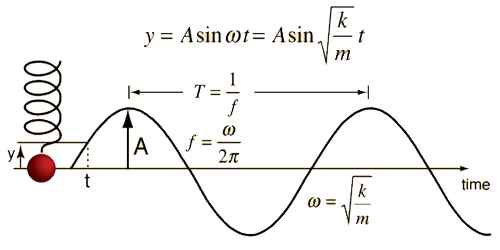Physics

# Differential Equation of the Simple Harmonic MotionDifferential Equation of the simple harmonic motion

Simple harmonic motion is produced due to the oscillation of a spring. Find out the differential equation for this simple harmonic motion. Suppose mass of a particle executing simple harmonic motion is ‘m’ and if at any moment its displacement and acceleration are respectively x and a, then according to definition,

a = – (K/m) x, K is the force constant.

But a = d2x/dt2

So, d2x/dt2 = – (K/m) x … … … (1)

In order to solve any differential equation, a general procedure is to assume a solution and it is observed whether the given differential equation can be derived from it or not. Suppose the solution of the equation (1) is –

x(t) = a sin ωt, here a and ω are constants.

dx/dt = a ω cos ωt and d2x/dt2 = – a ω2 sin ωt

From equation we get,

– a ω2 sin ωt = – (K/m) a sin ωt

So, if the value of the constant is, ω = √(K/m) … … … (2)

then, the relation x (t) = a sin ωt satisfies the differential equation.

So, a solution of the equation is x(t) = a sin ωt.

Similarly, we can prove that the relation, x(t) = b cos ωt is also a solution. Hence, x(t) = a sin ωt or, x(t) = b cos ωt is the solution of the simple harmonic motion.

It can be proved that,

x(t) = a sin ωt + b cos ωt … … … (3)

This equation is the general solution of the differential equation (1), as,

dx/dt = a ω cos ωt – b ω sin ωt

or, d2x/dt2 = – a ω2 sin ωt – b ω2 cos ωt

= – ω2 (a sin ωt + b cos ωt) = – ω2x

Then, d2x/dt2 = – ω2x                (here = a sin ωt + b cos ωt)

So, d2x/dt2 + ω2x = 0 … … … (4)

So, equation (4) is the differential equation of the simple harmonic motion.